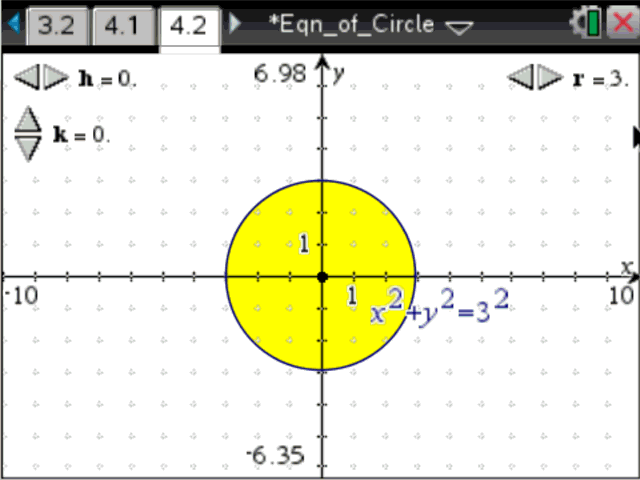# Activities

•• ##### Subject Area

• Standard: National Curriculum 7-10: Number and Algebra: Linear and non-Linear Relationships

• ##### AuthorMiddle

120 Minutes

• ##### Device
• TI-Nspire™
• TI-Nspire™ CAS
• TI-Nspire™ Navigator™
• ##### Software

TI-Nspire™
TI-Nspire™ CAS
TI-Nspire™ CAS Navigator™ NC System
TI-Nspire™ Navigator™ NC System

3.2

## Equation of a Circle#### Activity Overview

The aim of this investigation is to develop the equation of a circle of radius, r, centred at the origin, and to explore how the equation changes when the centre is translated parallel to the x and y axes.

#### Objectives

ACMNA267 - Describe, interpret and sketch parabolas, hyperbolas, circles and exponential functions and their transformations

• Circle
• Equation
• Translation
• Dilation

#### About the Lesson

In this activity, students explore the general equation of a circle with centre (h, k) and radius r. For the first part of the activity, students adjust the radius of the circle and drag a point around the circle to capture the coordinates. In the second part of the activity, students translate the circle using sliders for h and k to view how the equation changes.